## RD Sharma Class 8 Solutions Chapter 3 Squares and Square Roots Ex 3.9

These Solutions are part of RD Sharma Class 8 Solutions. Here we have given RD Sharma Class 8 Solutions Chapter 3 Squares and Square Roots Ex 3.9

Other Exercises

Using square root table, find the square roots of the following :

Question 1.
7
Solution: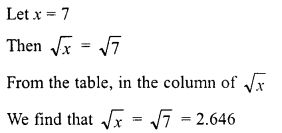Question 2.
15
Solution: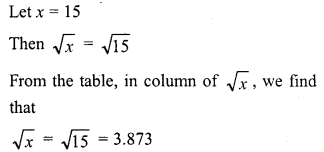Question 3.
74
Solution: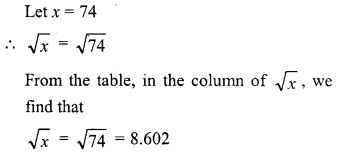Question 4.
82
Solution: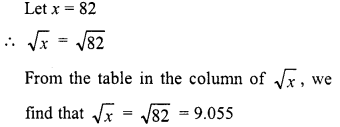Question 5.
198
Solution:Question 6.
540
Solution: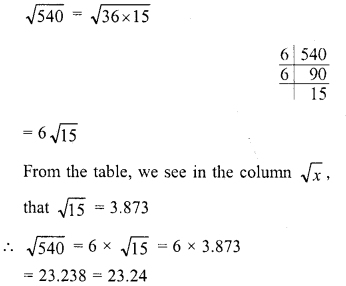Question 7.
8700
Solution: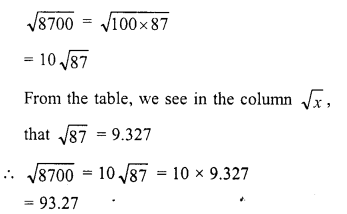Question 8.
3509
Solution: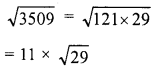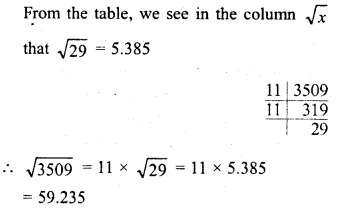Question 9.
6929
Solution: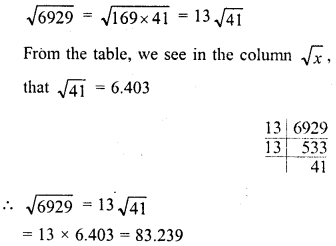Question 10.
25725
Solution:Question 11.
1312
Solution: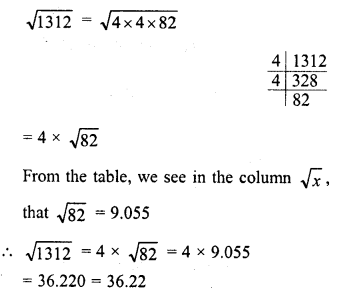Question 12.
4192
Solution: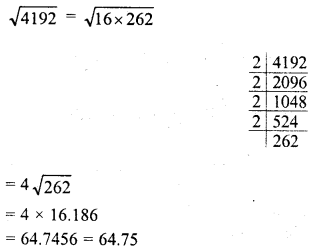Question 13.
4955
Solution:Question 14.
$$\frac {99 }{ 144 }$$
Solution: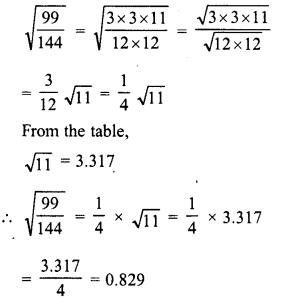Question 15.
$$\frac {57 }{ 169 }$$
Solution:Question 16.
$$\frac {101 }{ 169 }$$
Solution: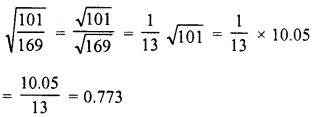Question 17.
13.21
Solution: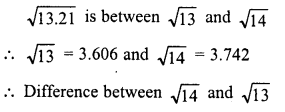Question 18.
21.97
Solution:Question 19.
110
Solution: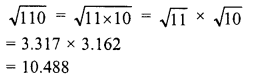Question 20.
1110
Solution: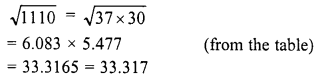Question 21.
11.11
Solution: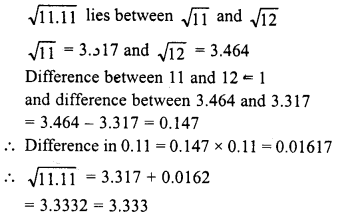Question 22.
The area of a square field is 325 m2. Find the approximate length of one side of the field.
Solution: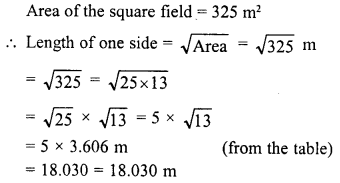Question 23.
Find the length of a side of a square, whose area is equal to the area bf the rectangle with sides 240 m and 70 m.
Solution: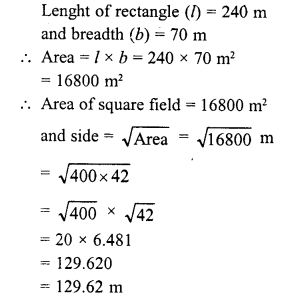Hope given RD Sharma Class 8 Solutions Chapter 3 Squares and Square Roots Ex 3.9 are helpful to complete your math homework.

If you have any doubts, please comment below. Learn Insta try to provide online math tutoring for you.